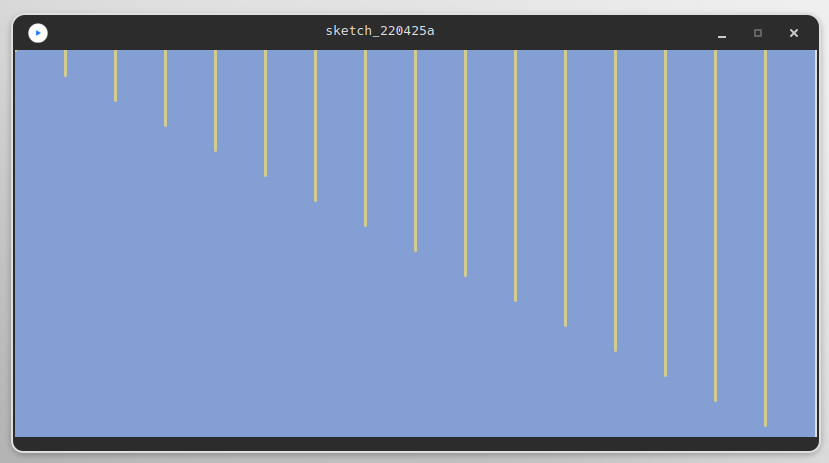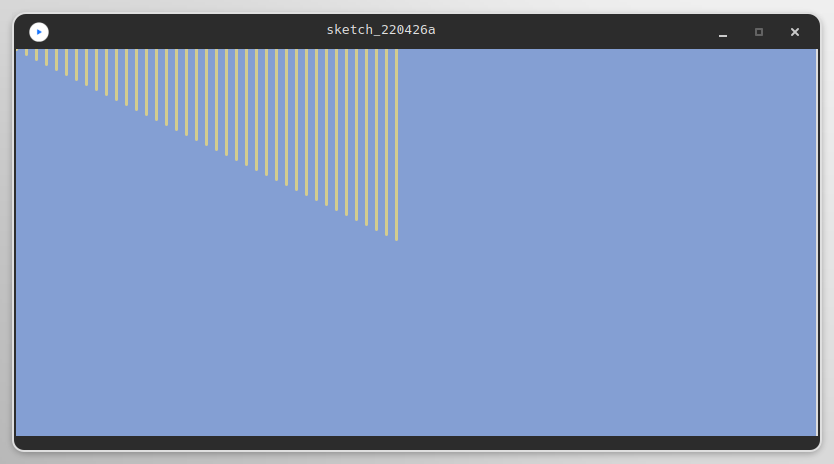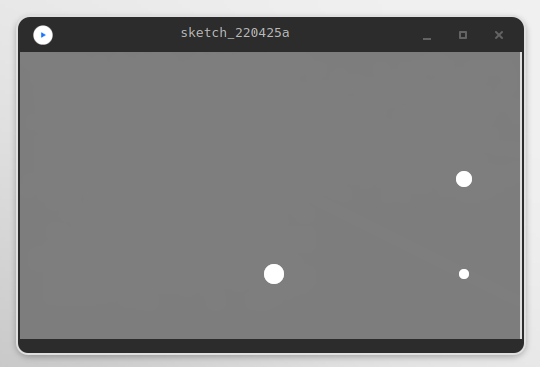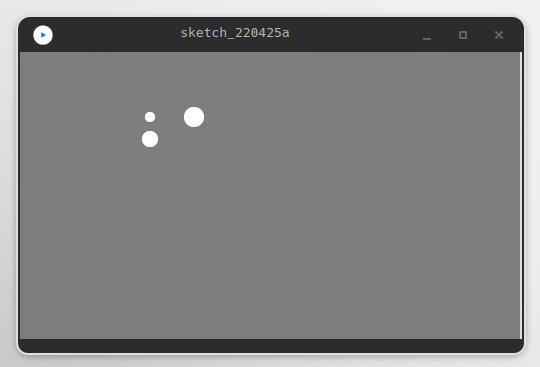# Control¶

## if … else if … else …¶

float r, g, b, a;

void setup() {
size(800, 500);
}

void draw() {
// Mouse in the left half:
if (mouseX < width/2) {
r = map(mouseX, 0, width/2, 0, 255);
}
// Mouse in the right half:
else {
g = map(mouseX, width/2, width, 0, 255);
}
// Mouse in the top half:
if (mouseY < height/2) {
b = map(mouseY, 0, height/2, 0, 255);
}
// Mouse in the bottom half:
else {
a = map(mouseY, height/2, height, 0, 255);
}

fill(r, g, b, a);
rect(0, 0, width, height);
}


Reference if

Reference else

## Relational operators¶

Relational operators compare two values and return a boolean value according to the operator.

Boolean is a simple data type which can have one of the two values true or false.

References:

== (equality)

!= (inequality)

< (less than)

<= (less than or equal)

>= (greater than or equal)

> (greater than)

These can be combined with so called logical operators:

&& (AND)

! (NOT)

|| (OR)

## Loops¶

### for-loop¶void setup() {
size(800, 400);
background(132, 159, 211);
stroke(211, 204, 143);
strokeWeight(3);

/* Loop from 0 to the width of the canvas
in steps of 50 pixels. */
for (int i = 0; i <= width; i += 50) {
// Draw a line from top to i/2 for every step.
line(i, 0, i, i/2);
}
}


### draw() - noLoop();¶/* Draw one line per frame. */
int i = 0;

void setup() {
size(800, 400);
background(132, 159, 211);
stroke(211, 204, 143);
strokeWeight(3);
frameRate(25);
}

void draw() {

line(i, 0, i, i/2);
i += 10;

if (i >= width) {
noLoop();
}
}


Instead of the for-loop from the previous example, draw is used to increase i. With if and noLoop() draw is paused when the loop is finished.

### draw - loop();¶

/* Draw one line per frame and reset the
loop on mousePressed(). */
int i = 0;

void setup() {
size(800, 400);
stroke(211, 204, 143);
strokeWeight(3);
frameRate(25);
}

void draw() {

if (i == 0) {
// set background/ clear canvas
background(132, 159, 211);
}

line(i, 0, i, i/2);
i += 10;

if (i >= width) {
// stop draw() when the end of the canvas is reached
noLoop();
}
}

void mousePressed() {
// reset i and start draw()
i = 0;
loop();
}


See redraw() as well.

## Irregular mappings¶

The previous sketches showed structures to control the program flow. Another level of control is the user interaction. Mappings provide control. If a user can easily identify the relation between user action and code action, it becomes predictable and the user is in control of the action./* Map the mouse position to the position of elements
in a non-regular relation. */

void setup() {
size(500, 300);
background(125);
noStroke();
noCursor();
}

void draw() {
// Background with some transparency.
fill(125, 100);
rect(0, 0, width, height);
// Color of the dots.
fill(255);
// Irregular mappings.
circle(mouseX, mouseX/2, 10);
circle(mouseY, mouseX/2, 20);
circle(mouseX, mouseY/2, 16);
}


After some interaction this irregular mapping is identified. What about switching mappings during runtime?